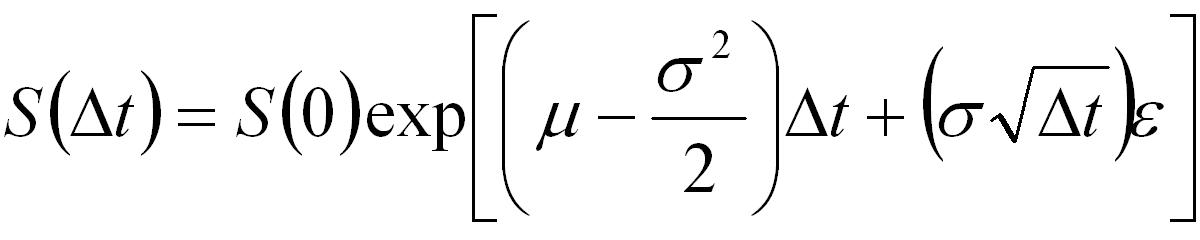## Using Control Variates in MATLAB

This tutorial presents MATLAB code that prices an Asian option using Monte-Carlo simulation in conjunction with the control variate variance reduction technique. For a general discussion of Monte-Carlo simulation see the Monte-Carlo Methods tutorial. And for a discussion of the mathematical background to control variates see the Variance Reduction tutorial.

Note that the primary purpose of the code presented here is to show how to implement the control variates technique and to price the option. The code contains no error checking and as such it is not suitable for inclusion into a larger application without appropriate modifications.

The Asian option under consideration has a payoff given byEquation 1: Payoff for an Asian Put and Call Option

where A is the average price of the underlying asset over the life of the option and X is the strike.

For the control variates technique the price of a similar option on the same asset should be known. In this case it is assumed that a plain vanilla Put and Call option is available that can be priced exactly using the Black-Scholes formula.

### MATLAB Script: AsianPutCallControlVariate

Assuming the underlying asset follows the standard log-normal/geometric Brownian motion model,Equation 2: Stock Price Evolution Equation

then the following MATLAB script gives an example of how to price an Asian option with payoff given by Equation 1. Note that this script calls the MATLAB function AssetPaths which is described in the Simulating Multiple Asset Paths in MATLAB tutorial.

```% Script to price an Put and Callon an Asian option using
% the Monte-Carlo approach with the control variate variance
% reduction technique

% Define the underlying parameters
S0 =50;
X = 50;
r = 0.04;
sig = 0.1;
dt = 1/365;
etime = 50; % days to expiry
T = dt*etime;

nruns = 100000;

% Calculate the Black-Scholes price for the European Call and Put
% This assumes that the Financial Toolbox is available
[blsEC,blsEP] = blsprice(S0,X,r,T,sig)

% Price the above vanilla Put and Call using Monte-Carlo
% The European option is not path dependent so only one step is required
S = AssetPaths(S0,r,sig,T,1,nruns);
Sf = S(end,:);  % The simulated prices at expiry
% Calculate the Monte-Carlo European Put and Call prices
mcEC = mean(max(Sf-X,0))*exp(-r*T)
mcEP = mean(max(X-Sf,0))*exp(-r*T)

% Calculate an initial price for the Asian option using Monte-Carlo
S = AssetPaths(S0,r,sig,dt,etime,nruns);
% calculate the payoff for each path for a Put
PutPayoffT = max(X-mean(S),0);
% calculate the payoff for each path for a Call
CallPayoffT = max(mean(S)-X,0);
% discount back
putPrice0 = mean(PutPayoffT)*exp(-r*T)
callPrice0 = mean(CallPayoffT)*exp(-r*T)

% Use the basic control variates approach to calculate a final price
putPrice = putPrice0 + (blsEP - mcEP)
callPrice = callPrice0 + (blsEC - mcEC)
```

### Example Usage

The result of executing the above script is,

```blsEC =
0.8809
blsEP =
0.6077
mcEC =
0.8784
mcEP =
0.6149
putPrice0 =
0.3573
callPrice0 =
0.4951
putPrice =
0.3501
callPrice =
0.4976
```

Note that the error in pricing the vanilla European option is used to (slightly) modify the price obtained using Monte-Carlo simulation without the control variate.

An example of pricing the same option without using antithetic variates is available in the Pricing an Asian Option in MATLAB tutorial. Other MATLAB based Monte-Carlo tutorials are linked off the Software Tutorials page.